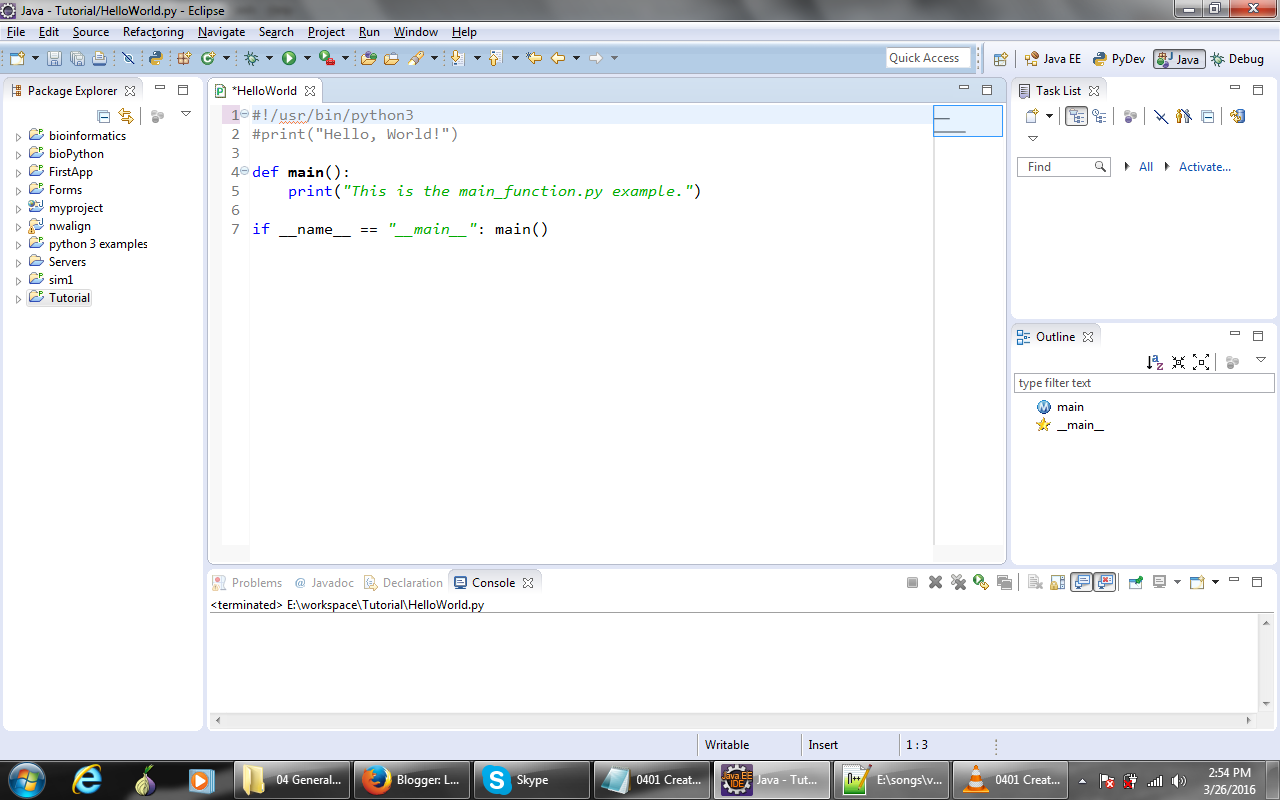Python General Syntax (comment, main function etc) - BOOKC -->

# Python General Syntax (comment, main function etc)

Monday, November 20, 2017

Hello how are you guys!!!, In my previous post (Python + Eclipe Development Environment / Python Hello World ) you learned about development environment of python and eclipse. You also learned how to create "Hello, World!" application in python. Today you will learn about common python syntax such as commenting code in python and how to create a main script in python.

Python Main Function:
In the below screen shot you will notice a few things. First of all, at the very top, there is this line with a Pound sign, which is the Comment Indicator in Python and an exclamation point and then a path to the Python Interpreter. This is used in environments that run the script from a shell, UNIX-based environments like a Mac or Linux environment, and one of the beauties of a scripting language like Python is that your script can run in a number of different environments without change.
The code of main function in python is given below:

`       `1. #!/usr/bin/python32. def main():3.    print("This is the main_function.py example.")4. if __name__ == "__main__": main()        ` `So it's important that you leave this as the first line of the file. Now this path here that starts with the first slash must be the path to the Python Interpreter. Now this is a very common path: /user/bin/ python3, that will work in a lot of environments. If you try to run this in UNIX- based environments and you find that it doesn't work, one thing that you'll want to look at is this path to see if the path needs to change.

Another thing that you will notice is this line (if __name__ == "__main__": main() ) here at the end. What this does is it allows us to run the script with the functions in any order that we want. Without this line and without the main execution of the script  being inside a function called main, then you wouldn't be able to run functions that are defined after the function is called.
In other words, if I put a function in the code below, and I say def example_function(): and print("example function") now I will need to call the example_function below of  this line, print("This is the main function.py example"), example_function().

def main():
print("This is the main function.py example.")
example_function()
def example_function():
print("example function")
if __name__ == "__main__": main()

Below is the screen shot of above code.